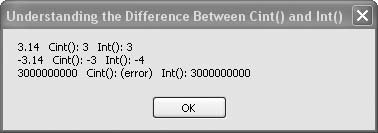# Recipe 6.20. Converting Numbers to Integers

#### Problem

You want to convert numbers to integers, or perhaps truncate or round values to integer values, and you want to understand the various ways to do this.

#### Solution

As always, use the best tool for the job. If you want to remove decimal parts of a number, consider using Int(), Floor(), or the Round() function. But if you want to convert a numeric value to an Integer data type, use CInt() or Convert.ToInteger() instead.

#### Discussion

The following code demonstrates differences between the CInt() and Int() functions. Once you gain a good understanding of these two functions, you'll be well on your way to understanding similar functions such as Round(), Convert.ToInteger(), and so on.

One important difference between CInt() and Int() is that Int() is overloaded to work with a wide variety of numeric data types. For example, you can pass a Double, such as the value of π, to Int(), and it will return another Double value that no longer has any post-decimal digits (i.e., it will round to a whole number). This is entirely different from converting a number to an Integer. The Int() function works on numbers that are way out of the legal range for an Integer. Using CInt() on similar numbers would throw an exception.

The two functions are demonstrated in the following code:

` Dim result As New System.Text.StringBuilder Dim number As Double ' ----- Positive decimal value. number = 3.14 result.Append(number) result.Append("   CInt(): ") result.Append(CInt(number).ToString) result.Append("   Int(): ") result.AppendLine(Int(number).ToString) ' ----- Negative decimal value. number = -3.14 result.Append(number) result.Append("   CInt(): ") result.Append(CInt(number).ToString) result.Append("   Int(): ") result.AppendLine(Int(number).ToString) ' ----- Number that won't fit in an Integer. number = 3000000000.0 result.Append(number) result.Append("   CInt(): ") Try     result.Append(CInt(number).ToString) Catch     result.Append("(error)") End Try result.Append("   Int(): ") result.Append(Int(number).ToString) MsgBox(result.ToString())  `

There are some other functions in the Math object that provide similar functionality to Int(). For example, the Math.Floor() and Math.Ceiling() functions also operate on numbers that might be out of the range of Integers. Floor() returns the largest whole number less than or equal to a given number, and Ceiling() returns the smallest whole number that's greater than or equal to a given number. See Figure 6-20.

##### Figure 6-20. The CInt( ) function converts numbers to Integer data types, while the Int( ) function returns whole numbersVisual Basic 2005 Cookbook: Solutions for VB 2005 Programmers (Cookbooks (OReilly))
ISBN: 0596101775
EAN: 2147483647
Year: 2006
Pages: 400

Similar book on Amazon